[[d.project_title]][[d.material_name]]# 我的麦昆有慧眼1x

1x
Pixy CMUcam5 图像识别传感器
2x
180° 微型舵机
1x

1x
3节5号电池盒（改装）
3x
3200mA 1.5V 5号电池
12x

“Pixy是一个开源的图像识别传感器，支持多物体，多色彩的颜色识别，最高支持7种颜色。你可以告诉它你想要的颜色，教它找东西。Pixy支持多种通信方式，如SPI，I2C等，可以直插在Arduino控制板上面。把它安装到你的机器人上，为你的小机器人增加一副眼睛。它搭载的图像传感器配合强大的硬件，可以配合PC跟踪、分析多色的数据。 Pixy是由Charmed实验室联合卡内基梅隆大学共同推出的一款图像传感器。其强大的处理器上搭载着一个图像传感器，它会选择性的处理有用的信息，因为它采用以颜色为中心的办法——使该产品只是将特定颜色的物体的视觉数据发送给相互配合的微型控制器，而不是输出所有视觉数据以进行图像处理。所以你的Arduino板或其它微控制器很容易跟它交流，并滕出许多CPU来执行其他事件。”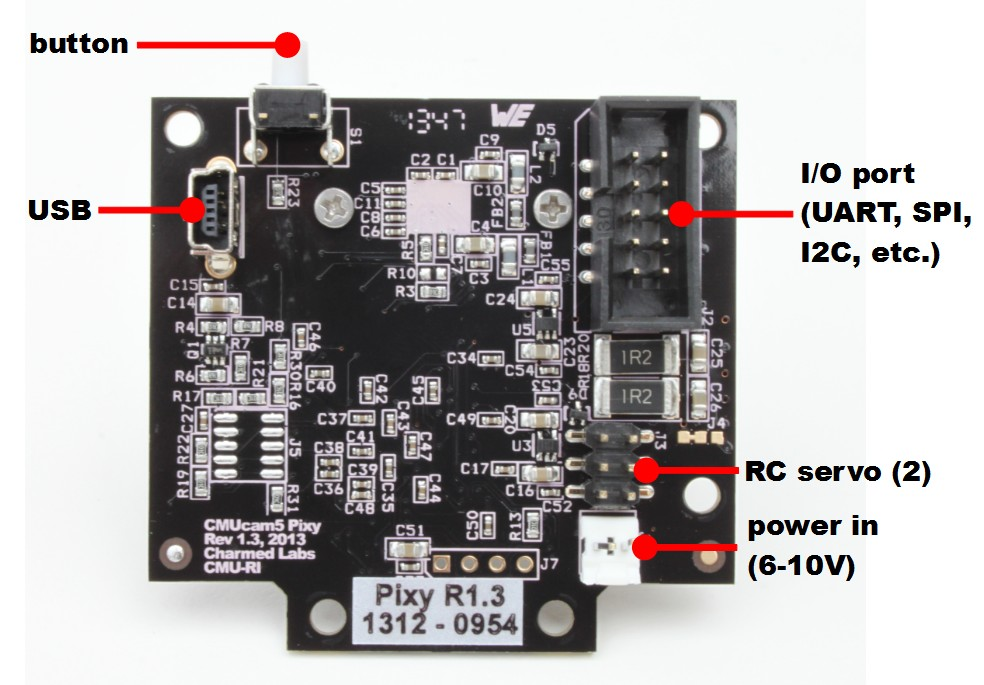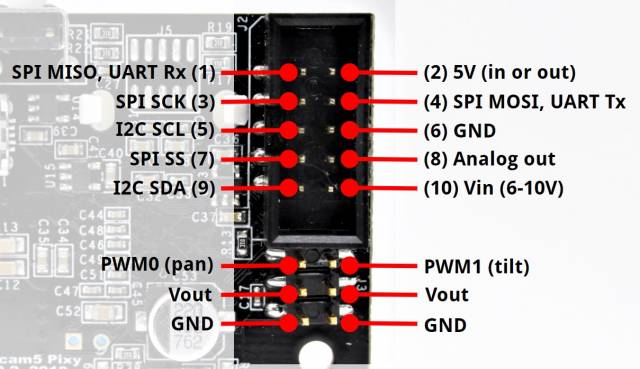I / O端口的引脚按以下顺序排列，左上角是引脚1，右下角是引脚10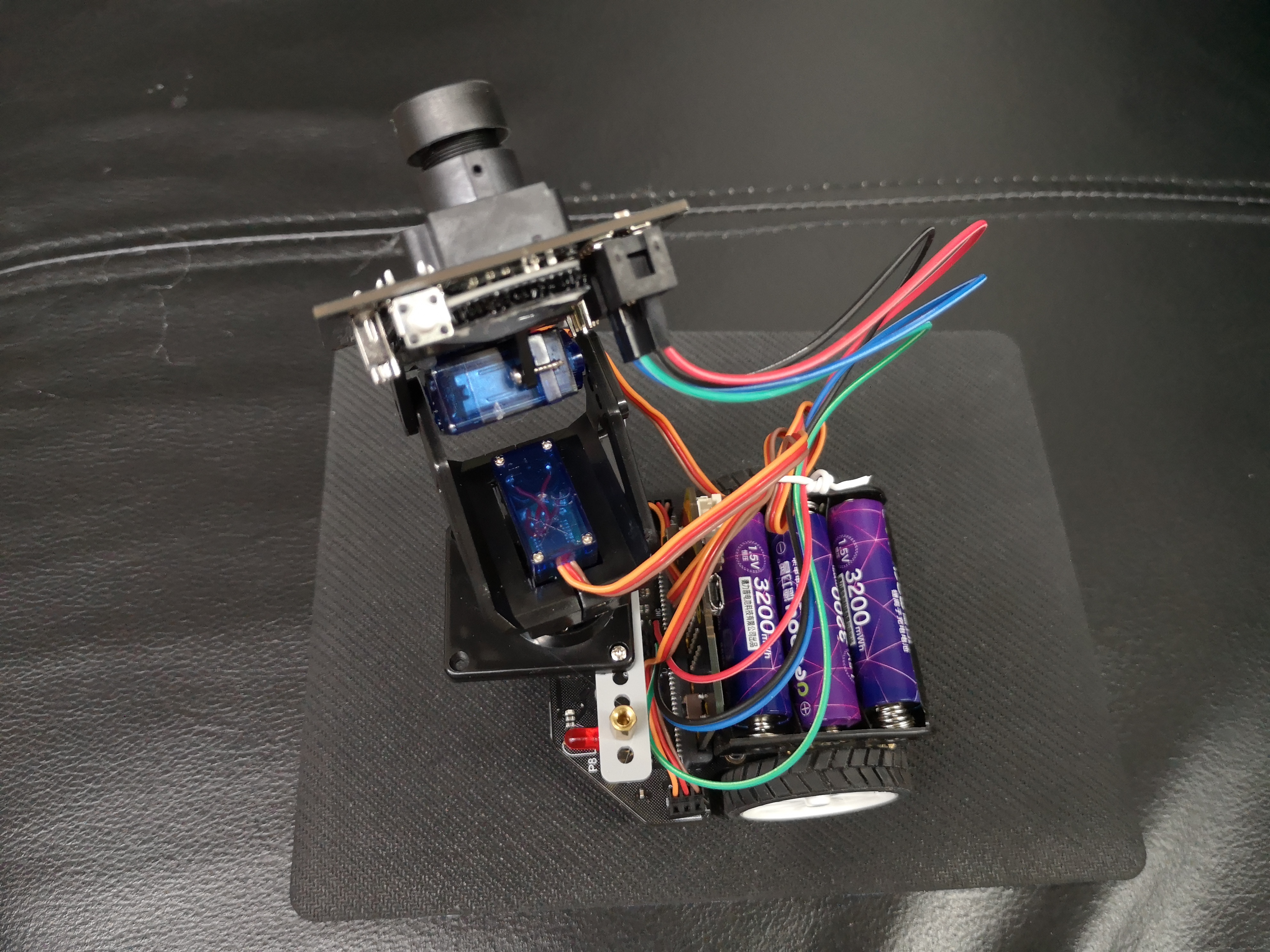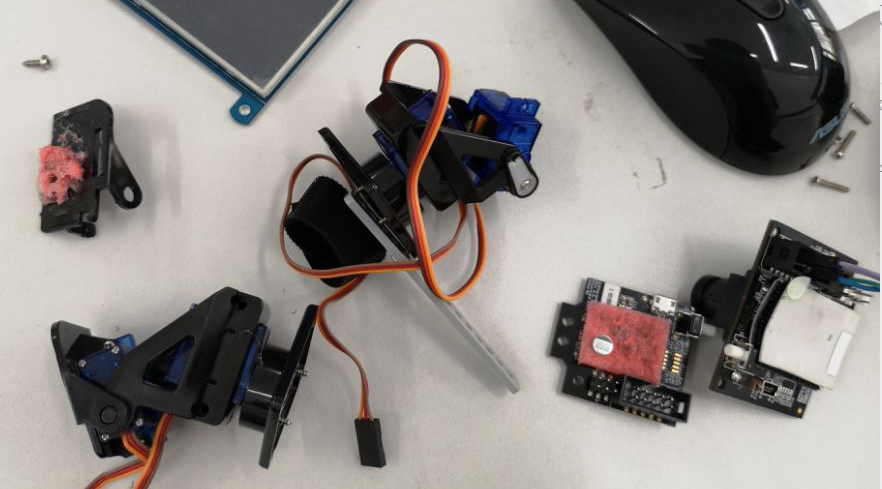Makecode

#### 编程语言

Javascript

makecode中我也是大部分选择代码的方式，因为写代码比拖动积木块高效，而且makecode的代码补全、语法错误检查还是非常好用的。最重要的一点是程序使用了类以及带参数的函数，积木块是无法实现这些功能的。

##### 代码：定义变量

```	                    					```//变量定义
let PIXY_START_WORD_CC = 0
let oldX = 0
let oldY = 0
let oldSignature = 0
let Y_CENTER = 0
let newSize = 0
let X_CENTER = 0
let maxSize = 0
let obj_Y = 0
let obj_X = 0
let signature = 0
let checkSum = 0
let lastBlockTime = 0
let PIXY_START_WORD = 0
let var2 = 0
let var1 = 0
let rightSpeed = 0
let leftSpeed = 0
let height = 0
let width = 0
let tiltError = 0
let panError = 0
let RCS_CENTER_POS = 0
let RCS_MAX_POS = 0
let RCS_MIN_POS = 0
let size = 0```
```

##### 代码：定义函数用于跟随物体行走

```	                    					```// 定义函数用于跟随物体行走
function FollowBlock(width: number, height: number) {
let followError = 0
let forwardSpeed = 0
let differential = 0
followError = RCS_CENTER_POS - panLoop.m_pos
//basic.showString("E:" + Math.trunc(followError))
size += width * height
//basic.showString("S:" + Math.trunc(size))
// size -= size / 8
forwardSpeed = Math.constrain(400 - (size / 256), -100, 400) / 10
//basic.showString("F:" + Math.trunc(forwardSpeed))
differential = (followError + (followError * forwardSpeed)) / 256
//basic.showString("D:" + Math.trunc(differential))
leftSpeed = Math.constrain(forwardSpeed + differential, -400, 400) / 10
//basic.showString("L:" + Math.trunc(leftSpeed))
rightSpeed = Math.constrain(forwardSpeed - differential, -400, 400) / 10
//basic.showString("R:" + Math.trunc(rightSpeed))
maqueen.MotorRun(maqueen.aMotors.M1, maqueen.Dir.CW, leftSpeed)
maqueen.MotorRun(maqueen.aMotors.M2, maqueen.Dir.CW, rightSpeed)
}```
```

##### 代码：初始化云台位置，初始化变量

```	                    					```// 初始化摄像头云台位置
pins.servoWritePin(AnalogPin.P1, 90)
pins.servoWritePin(AnalogPin.P2, 90)
// 变量初始化
maxSize = 0
newSize = 0
oldSignature = 0
oldY = 0
oldX = 0
width = 0
height = 0
oldSignature = 0
X_CENTER = 160
Y_CENTER = 100
RCS_MIN_POS = 0
RCS_MAX_POS = 180
size = 400
RCS_CENTER_POS = (RCS_MAX_POS - RCS_MIN_POS) / 2
PIXY_START_WORD = -21931
PIXY_START_WORD_CC = -21930
lastBlockTime = 0
maqueen.motorStopAll()```
```

```	                    					```#define PIXY_ARRAYSIZE              100
#define PIXY_START_WORD             0xaa55
#define PIXY_START_WORD_CC          0xaa56
#define PIXY_START_WORDX            0x55aa
#define PIXY_SERVO_SYNC             0xff
#define PIXY_CAM_BRIGHTNESS_SYNC    0xfe
#define PIXY_LED_SYNC               0xfd
#define PIXY_OUTBUF_SIZE            64```
```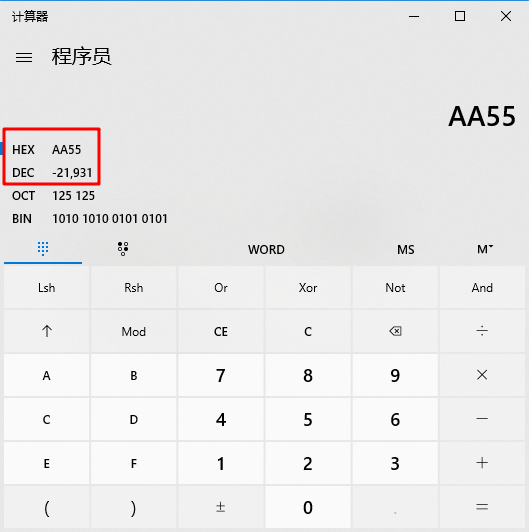##### 代码：定义类及实例化

```	                    					```class ServoLoop {

m_pos: number

m_prevError: number

m_proportionalGain: number

m_deritativeGain: number

constructor(propotionalGain: number, derivativeGain: number) {

this.m_pos = RCS_CENTER_POS

this.m_proportionalGain = propotionalGain

this.m_deritativeGain = derivativeGain

this.m_prevError = 0x80000000

}

update(error: number) {

let velocity = 0

if (this.m_prevError != 0x80000000) {

velocity = (error * this.m_proportionalGain + (error - this.m_prevError) * this.m_deritativeGain) / 1024

this.m_pos += velocity

if (this.m_pos > RCS_MAX_POS) {

this.m_pos = RCS_MAX_POS

}

else if (this.m_pos < RCS_MIN_POS) {

this.m_pos = RCS_MIN_POS

}

}

this.m_prevError = error

}

}
let panLoop = new ServoLoop(5, 200)
let tiltLoop = new ServoLoop(15, 200)```
```

##### 代码：程序主体

```	                    					```basic.forever(function () {
if (var1 != 0) {
if (var1 == PIXY_START_WORD) {
if (var2 == PIXY_START_WORD) {
panError = X_CENTER - obj_X
tiltError = obj_Y - Y_CENTER
panLoop.update(panError)
tiltLoop.update(tiltError)
pins.servoWritePin(AnalogPin.P1, panLoop.m_pos)
pins.servoWritePin(AnalogPin.P2, tiltLoop.m_pos)
FollowBlock(width, height)
oldX = obj_X
oldY = obj_Y
oldSignature = signature
}
}
}
})```
```
##### 关于PID算法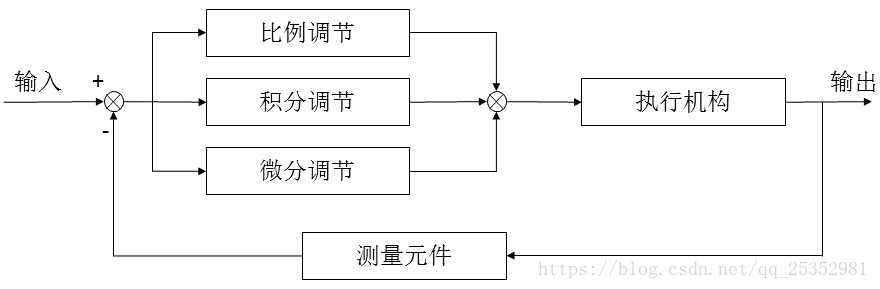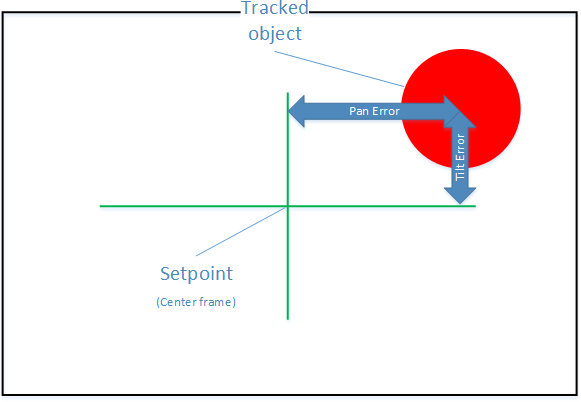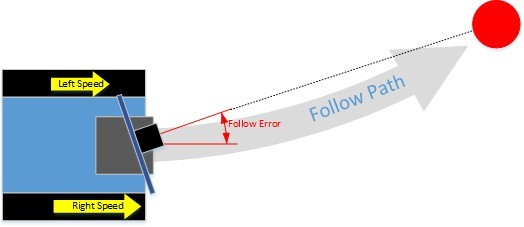#### 参考资料

Makelog作者原创文章，未经授权禁止转载。
13
8[[c.user_name]] [[c.create_time]]
[[c.parent_comment.count]]
|
[[c.comment_content]]
[[r.user.user_name]] [[r.create_time]]
[[r.comment_content]]

1
1
7
15
5
15
10
13
0
1